Using the Fourier Transform to Solve the Harmonic Oscillator and Back-Transforming

All the derivatives have been removed, and we can solve for the Fourier transformed as a function of the transformation variable (in this case, ω).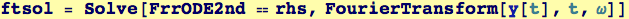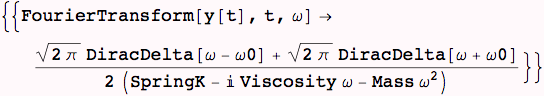Build in some physical assumptions for mass-spring-dashpot systems.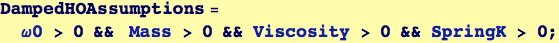Back-transform to find the solution.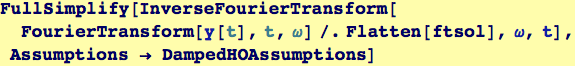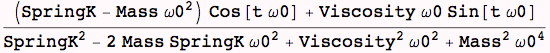Otherwise, the complete solution (i.e., the inhomogeneous plus the homogeneous parts) can be calculated by the DSolve function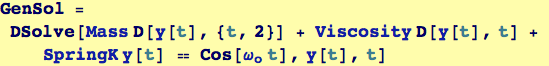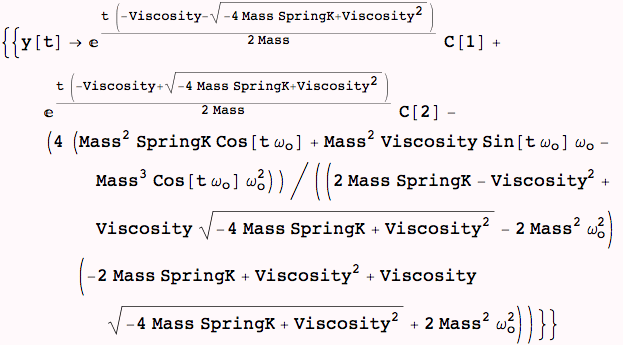Created by Wolfram Mathematica 6.0  (26 November 2007)